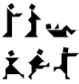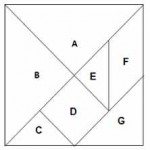This is a classic Chinese Tangram. Each of the people illustrated, and many convex polygons, can be made with the 7 pieces and there are hundreds of other puzzles based on this tangram.

Can you find a way to fold a sheet of paper so that you can cut out the tangram pieces accurately without any measuring?

Take the edge length of the square as 1 unit.

Find the areas of the 7 pieces. What fraction of the square does each piece make?

Make a square from the pieces A, G, C and E. What is its area? What is its edge length?

How many different polygons can you make using all 7 pieces of the tangram?

AIMSSEC Teacher Notes TANGRAM FRACTIONS
For a bit of fun see http://nrich.maths.org/1491

Tagged with:

### 4 Responses to Grades 6 to 10 Tangram Fractions

1.Maitse Phooko says:

We started by making tangram squares with papers with my grade 6 learners. Each learner had his or her own pieces. They discovered which shapes were the same. I asked them to make a square and a triangle from shapes A,G,C,E. They managed to do the shapes very fast.

2.Ntholeng Lekhetha says:

First of all I thank this course in teaching us how to make our 7 pieces, it is easy and cheap. I did this in Grade 5, Grade 6 and Grade 7. They enjoyed the tangrams although it was not easy for them to do the shapes but it was very difficult for them to give-up. Others were saying:’No teacher this is impossible we cant make the shapes with this pieces’.

3.Ntholeng Lekhetha says:

Many learners enjoy the tangrams, they did many shapes although there are those that are very difficult for them.

4.toni says:

To clear up some confusion about what the word CONVEX means when we talk about convex polygons, imagine you are an ant crawling around the edges of a polygon. let’s say you are going around clockwise then, if you turn right at EVERY vertex until you get back to facing the way you set out from your starting point, then the polygon is convex and all the interior angles are less than 180 degrees. If you turned left at any vertex then that angle was greater than 180 degrees and the polygon was not convex.

This method also shows that the SUM OF THE EXTERIOR ANGLES OF ANY CONVEX POLYGON is 360 degrees because the ant must turn through a total of exactly 360 degrees on his way round.

If the polygon is REGULAR with n edges and vertices then each exterior angle will be 360/n degrees and each interior angle will be (180 – 360/n) degrees. For example the formulas give exterior angles of 72 degrees for n=5 and interior angles of 108 degrees.